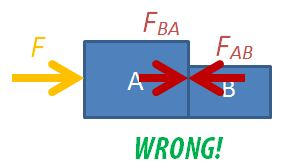# 03 Dynamics

## Pendulum-Powered Car

This pendulum-powered car is constructed using Lego Technic parts. I used mainly Lego beams to create the chassis and an “A” frame from which the pendulum is suspended. The pendulum is made of Lego beams and some wheels.

When the pendulum swings, it experiences an acceleration towards its equilibrium position. By the principle of conservation of momentum, the car experiences a change in momentum in the opposite direction. Since the acceleration of the pendulum changes its direction every half a cycle of its oscillation, the car will only oscillate about its original position if the wheels of the car are free to turn throughout the oscillation.

A escapement mechanism which consists of a beam resting on a pair of 40-tooth gears attached to the front wheels prevent the wheels from rotating in the opposite direction. This means that the car will only be moving forward during the half of the pendulum’s oscillation when its displacement is at the front of its equilibrium position and pauses during the other half.## Free-Body Diagrams in Two-Body Motion

Students are often confused about the forces in drawing free-body diagrams, especially so when they have to consider the different parts of multiple bodies in motion.

##### Two-Body Motion

Let’s consider the case of a two-body problem, where, a force F is applied to push two boxes horizontally. If we were to consider the free-body diagram of the two boxes as a single system, we only need to draw it like this.

For the sake of problem solving, there is no need to draw the normal forces or weights since they cancel each other out, so the diagram can look neater. Applying Newton’s 2nd law of motion, $F=(m_A+m_B) \times a$, where $m_A$ is the mass of box A, $m_B$ is the mass of box B, F is the force applied on the system and a is the acceleration of both boxes.

You may also consider box A on its own.

The equation is $F-F_{AB}=m_A \times a$, where $F_{AB}$ is the force exerted on box A by box B.

The third option is to consider box B on its own.

The equation is $F_{BA}=m_B \times a$, where $F_{BA}$ is the force exerted on box B by box A. Applying Newton’s 3rd law, $F_{BA}=F_{AB}$ in magnitude.

##### Never Draw Everything Together

NEVER draw the free-body diagram with all the forces and moving objects in the same diagram, like this:You will not be able to decide which forces acting on which body and much less be able to form a sensible equation of motion.

Interactive

Use the following app to observe the changes in the forces considered in the 3 different scenarios. You can vary the masses of the bodies or the external force applied.

##### Multiple-Body Problems

For the two-body problem above, we can consider 3 different free-body diagrams.

For three bodies in motion together, we can consider up to 6 different free-body diagrams: the 3 objects independently, 2 objects at a go, and all 3 together. Find the force between any two bodies by simplifying a 3-body diagram into 2 bodies. This trick can be applied to problems with even more bodies.

## Collision Simulation

I created this post here to bookmark some useful tools for use during my upcoming JC1 lectures on Dynamics.

This is a simulation for collisions that show the momenta before and after collisions. It requires registration after one visit.

A better choice for now could be the EJSS version (created by my ex-colleague Lawrence) which is far more detailed.

I had wanted to build one using GeoGebra and in fact, was halfway through it, but the Covid-19 pandemic has created other areas of work that now take priority.

## Two Body Problems in Dynamics

Problems involving two bodies moving together usually involve asking for the magnitude of the force between the two.

For example:

A 1.0 kg and a 2.0 kg box are touching each other. A 12 N horizontal force is applied to the 2.0 kg box in order to accelerate both boxes across the floor. Ignoring friction, determine:

(a) the acceleration of the boxes, and

(b) the force acting between the boxes.

To solve for (b) requires an understanding that the free-body diagram of the 1.0 kg box can be considered independently as only the force acting between the two boxes contributes to its acceleration since it is the only force acting on it in the horizontal direction.

This interactive app allows for students to visualise the forces acting on the boxes separately as well as a single system.

The codes for embedding into SLS:

<iframe scrolling="no" title="Two Mass Problem" src="https://www.geogebra.org/material/iframe/id/fh5pwc37/width/638/height/478/border/888888/sfsb/true/smb/false/stb/false/stbh/false/ai/false/asb/false/sri/false/rc/false/ld/false/sdz/false/ctl/false" width="638px" height="478px" style="border:0px;"> </iframe>

## Box on a Slope Simulation

This simulation allows students to observe the variation of the normal contact force N acting on a box placed on a frictionless slope with the angle of inclination of the slope changing. It also allows them to see how the Weight vector W can be resolved into two components, with the one perpendicular to the slope being equal at all times to N. Meanwhile, the component of W parallel to the slope being proportional and in the same direction as acceleration.